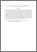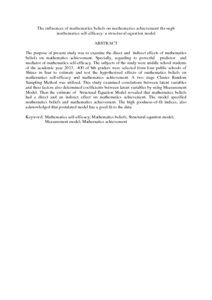# The influences of mathematics beliefs on mathematics achievement through mathematics self-efficacy: a structural equation model

## Citation

Kamalimoghaddam, Hajar and Ahmad Tarmizi, Rohani and Mohd Ayub, Ahmad Fauzi and Wan Jaafar, Wan Marzuki (2016) The influences of mathematics beliefs on mathematics achievement through mathematics self-efficacy: a structural equation model. Communication in Mathematical Modeling and Applications, 1 (1). pp. 44-51. ISSN ESSN: 2149-7168

## Abstract

The purpose of present study was to examine the direct and indirect effects of mathematics beliefs on mathematics achievement. Specially, regarding to powerful predictor and mediator of mathematics self-efficacy. The subjects of the study were middle school students of the academic year 2013. 400 of 8th graders were selected from four public schools of Shiraz in Iran to estimate and test the hypothesized effects of mathematics beliefs on mathematics self-efficacy and mathematics achievement. A two stage Cluster Random Sampling Method was utilized. This study examined correlations between latent variables and their factors also determined coefficients between latent variables by using Measurement Model. Then the estimate of Structural Equation Model revealed that mathematics beliefs had a direct and an indirect effect on mathematics achievement. The model specified mathematics beliefs and mathematics achievement. The high goodness-of-fit indices, also acknowledged that postulated model has a good fit to the data.Preview
PDF (Abstract)
The influences of mathematics beliefs on mathematics achievement through mathematics self-efficacy a structural equation model.pdfView Item